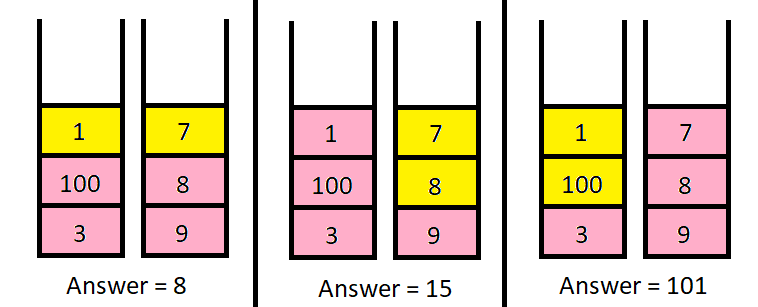Formatted question description: https://leetcode.ca/all/2218.html

# 2218. Maximum Value of K Coins From Piles (Hard)

There are n piles of coins on a table. Each pile consists of a positive number of coins of assorted denominations.

In one move, you can choose any coin on top of any pile, remove it, and add it to your wallet.

Given a list piles, where piles[i] is a list of integers denoting the composition of the ith pile from top to bottom, and a positive integer k, return the maximum total value of coins you can have in your wallet if you choose exactly k coins optimally.

Example 1:Input: piles = [[1,100,3],[7,8,9]], k = 2
Output: 101
Explanation:
The above diagram shows the different ways we can choose k coins.
The maximum total we can obtain is 101.


Example 2:

Input: piles = [,,,,,,[1,1,1,1,1,1,700]], k = 7
Output: 706
Explanation:
The maximum total can be obtained if we choose all coins from the last pile.


Constraints:

• n == piles.length
• 1 <= n <= 1000
• 1 <= piles[i][j] <= 105
• 1 <= k <= sum(piles[i].length) <= 2000

Companies:

Related Topics:
Array, Dynamic Programming, Prefix Sum

Similar Questions:

## Solution 1. Top-down DP

Let dp[i][j] be the max value of j coins using piles i ~ N-1. The answer is dp[k].

For dp[i][j], we can try using t elements from A[i] (0 <= t <= min(j, A[i].size())), getting A[i] + ... + A[i][t-1] value plus dp[i+1][j-t] value (the max value of j-t coins using piles i+1 ~ N-1). We try different ts and assign the max value to dp[i][j].

dp[i][j] = max( dp[i+1][j-t] + sum(i, t) | 0 <= t <= min(j, A[i].size()) )
where sum(i, t) = A[i] + ... + A[i][t-1]


The trivial case is dp[N][j] = 0, i.e. we can’t get any value from the nonexistent A[N].

// OJ: https://leetcode.com/problems/maximum-value-of-k-coins-from-piles/
// Time: O(NM) where M = sum(A[i].size())
// Space: O(NK)
class Solution {
public:
int maxValueOfCoins(vector<vector<int>>& A, int k) {
int N = A.size(), m = {};
memset(m, -1, sizeof(m));
function<int(int, int)> dp =[&](int i, int j) {
if (i == N) return 0;
if (m[i][j] != -1) return m[i][j];
int ans = dp(i + 1, j), sum = 0;
for (int t = 1; t <= j && t <= A[i].size(); ++t) {
sum += A[i][t - 1];
ans = max(ans, dp(i + 1, j - t) + sum);
}
return m[i][j] = ans;
};
return dp(0, k);
}
};


## Solution 2. Bottom-up DP

// OJ: https://leetcode.com/problems/maximum-value-of-k-coins-from-piles/
// Time: O(NK)
// Space: O(NK)
class Solution {
public:
int maxValueOfCoins(vector<vector<int>>& A, int k) {
int dp = {}, N = A.size();
for (int i = 0; i < N; ++i) {
for (int j = 1; j <= k; ++j) {
dp[i + 1][j] = dp[i][j];
for (int t = 1, sum = 0; t <= j && t <= A[i].size(); ++t) {
sum += A[i][t - 1];
dp[i + 1][j] = max(dp[i + 1][j], dp[i][j - t] + sum);
}
}
}
return dp[N][k];
}
};


The space complexity can be reduced to O(K).

// OJ: https://leetcode.com/problems/maximum-value-of-k-coins-from-piles/
// Time: O(NK)
// Space: O(K)
class Solution {
public:
int maxValueOfCoins(vector<vector<int>>& A, int k) {
int dp = {}, N = A.size();
for (int i = 0; i < N; ++i) {
for (int j = k; j >= 1; --j) {
for (int t = 1, sum = 0; t <= j && t <= A[i].size(); ++t) {
sum += A[i][t - 1];
dp[j] = max(dp[j], dp[j - t] + sum);
}
}
}
return dp[k];
}
};


## Discuss

https://leetcode.com/problems/maximum-value-of-k-coins-from-piles/discuss/1886910